Article byExcelMojo Team## What Is Add Time In Excel?

Add time in Excel is an option that enables one to add time values, hours, minutes, and seconds to a given time value. And we can use the ‘+’ operator or Excel inbuilt function SUM to add time values in a spreadsheet.

Users can add time values in Excel when working on aspects such as calculating the total time to complete a project based on each phase time.

For example, the table below shows the time taken for a job applicant to complete two interview rounds.

And the requirement is to calculate the total time the applicant takes to complete the interview sessions and display the output in cell B5.

Then, we can use the SUM() based add time in Excel formula in the target cell to fetch the required data.

The above scenario is an add time in Excel hours and minutes example, where the SUM() adds the time values specified in cells B2 and B3, 2:30 and 1:30. And the function returns the sum of two and a half and one and half hours, which is 4:00 or four hours in the target cell.

In the backend, Excel adds the decimal equivalents of the time values and sets the default time format for the specific cell to display the required total time value.

###### Key Takeaways
• Add time in Excel, sum up time values, and add under or over 23 hours, 59 minutes, or 59 seconds to time values.
• Users can add time values in Excel when performing time-based calculations, such as finding the total duration of running a software application involving multiple stages.
• Also, we can use the ‘+’ operator or the SUM function to add time values in Excel.
• We can use the Custom category in the Number tab in the Format Cells window to customize and set the required time format in the required cells.

### How To Add Time In Excel?

The steps to apply the add time in Excel formula to find the sum of time values are as follows:

1. First, prepare the source dataset by listing the time values we must add.
2. Next, choose a target cell, enter the ‘=‘ symbol, and the cell reference to each time value in the source dataset alternatively with the ‘+’ operator.

[Alternatively, choose a target cell and enter the SUM() directly, with the cells or cell range references containing the time values.

And if the time values to add are in contiguous cells in the source data. Then, we can select a cell below the last cell with the time value. And then, choose Home or Formulas AutoSum to view the SUM() with the required source cell range in the target cell. And, if required, we can change the cell range within the inserted SUM() to fit our time value addition requirements.]

1. Then, press Enter to view the required total time value in the target cell.

The steps will fetch us the required output. However, the output may appear incorrect if the total time value exceeds 24 hours. Thus, in such scenarios, we must follow the below additional steps.

1. To begin with, choose the target cell containing the formula to automatically add time in Excel and then, select the Home tab → Number FormatMore Number Formats.

The above step will open the Number tabin the Format Cells window.

[Alternatively, right-click the target cell, choose Format Cells from the contextual menu or press Ctrl + 1 to access the Format Cells window.]

1. Next, select the Custom category from the Category list on the left in the Number tab. And then, enter the time format, “[h]:mm;@”, in the Type field and click OK to add the format to the Custom formats list.

The above step will show the correct total time value exceeding 24 hours in the specified time format in the target cell.

### Examples

The following illustrations explain the methods to automatically add time in Excel.

#### Example #1

The table below lists activities conducted in a program and their durations.

And the requirement is, to sum up the given time values to display as the total program duration in cell C7.

Then, as the time values in the source data show hours and minutes with seconds being zero, the scenario is an add time in Excel hours and minutes example. And the steps to add the time values and show the required outcome in the target cell are as follows:

• Step 1: To start with, choose cell C7 and then, enter the following formula.

=C3+C4+C5+C6

• Step 2: Then, press Enter to execute the formula and view the value of 11:10 as the required total program duration in cell C7.

[ Alternatively, we can choose cell C7 and enter the SUM(), as  shown below:

=SUM(

Next, enter the cell range, C3:C6, containing the given time values to add.

=SUM(C3:C6

And then, close the bracket to complete the formula.

Next, press Enter to execute the SUM().

Alternatively, we can choose cell C7 and Formulas Math & Trig SUM.

Now, the Function Arguments window will open. And, since we have the target cell just below the source data set range, the first field in the window shows the required cell range selected.

Also, we do not have any further time values to add. So we do not require to update the subsequent fields in the window.

Next, click OK to execute the SUM().

Meanwhile, we can select cell C7 and then, click Home or Formulas AutoSum.

Now, the SUM() with the data range right above the chosen cell gets inserted in the target cell.

And as the highlighted cell range covers the required time values, we can press Enter to view the function output.]

Now, adding the given time values, 3:15, 4:10, 1:45, and 2:00, will sum the minutes, which is 70. But 60 minutes make an hour. So, out of the 70 minutes, 60 minutes add to the hour values, showing the remaining minutes as the final total minutes, 10. And the hour values add with the carry-over value of 1, making the total hours 11.

Excel adds the decimal equivalents of the time values and sets the default time format for the specific cell to display the required total time value.

#### Example #2

In the previous example, the total program duration is within 24 hours. And hence, we obtained the required total time without modifying the output’s time format.

We shall see what happens when adding time values leads to over 24 hours.

The table below shows the time an employee worked in two shifts.

And the requirement is to add the given time values to obtain the total time the employee worked across the two shifts in cell D7. Then, we can apply the SUM() in the target cell to fetch the required data, as explained below:

• Step 1: First, choose cell D7, enter the SUM(), and then, press Enter.

=SUM(D4:D5)

Though the time values provided as input are over 10 hours, the SUM() returns their sum as 2:15, which appears incorrect.

And selecting the time values in the source dataset will show the correct total time value at the bottom-right corner of the spreadsheet, 26:15:00.

It is so since the total of the specified time values is over 24 hours. And Excel divides the total 26:15 by 24 hours and displays the remaining 2:15 hours as the output.

So, to view the correct output, we can change the target cell format, as explained below:

• Step 2: Next, choose cell D7 and then, click HomeNumber Format More Number Formats to open the Format Cells window.

[Alternatively, select cell D7 and right-click to choose Format Cells from the contextual menu.

The above step is another way to access the Format Excel Cells window]

Now, the Number tab in the Format Cells window opens, showing cell D7’s current number format.

• Step 3: Next, enter the format “[h]:mm;@” in the field below the Type option.

And clicking OK in the Format Cells window will add the specified custom format to the existing list and show the correct output updated in the target cell.

[Alternatively, we can use the TEXT excel function with the SUM() and the above mentioned time format to achieve the required output.

In the above example, we chose cell D7, entered the TEXT() containing the SUM() and the required time format, and pressed Enter to obtain the correct total time value or 26:15]

The formula adds the decimal equivalents of the time values and sets the specified time format for the target cell to display the required total time value.

Theoretically, the minutes in the two specified time values get added. And we obtain a total of 75 minutes. Then, 60 out of the 75 minutes count as 1 hour, and the formula shows the final total minutes as 15. And the 1 hour gets carried over and added to the hours in the two specified time values, making the final total hours 26.

#### Example #3

The table below contains an initial time, 9:30 AM. And the second column shows the descriptions of the time values to add to the initial time value.

Assume we must add the time values according to the descriptions and display the output in cell range C3:C9.

And the steps are as follows:

• Step 1: To begin with, choose cell C3, enter the TIME excel function -based formula, and then, press Enter.

=A3+TIME(10,0,0)

The TIME() accepts three inputs, hour, minute, and second. And, since we must only add 10 hours to 9:30 AM, we supply the first argument value as 10 and the remaining as 0.

The TIME() output is 10:00. Then, the formula adds 10 hours to the hour part of the initial time value to give the result 19:30.

• Step 2: Next, select cell C4, enter the below formula, and then, press Enter.

=A3+25/24

The above formula is for adding over 24 hours to the initial time value. So, we divide the specified hours, 25, by 24 to get the remaining hour as 1 hour, which the formula adds to 9:30, making the final time 10:30.

However, adding 25 hours to 9:30 should be 34:30. So we must set the correct data format in cell C4, as explained in the previous example.

• Step 3: Now, select cell C4 and then, choose HomeNumber Format More Number Formats to access the Format Cells window.

And set the required custom time format using the option from the existing list.

And clicking OK will show the corrected final time value in cell C4.

Now, we shall see an add time in Excel minutes example.

• Step 4: Next, choose cell C5, enter the TIME()-based formula, and then, press Enter.

=A3+TIME(0,35,0)

Since we must add minutes to the initial time, the TIME() accepts the minute argument value, 35, with the remaining argument values being 0.

So, the TIME() output is 12:35 AM, which the formula adds to 9:30, resulting in the final time of 10:05 (22:05).

In the next add time in Excel minutes scenario, we shall add minutes over 59 minutes.

• Step 5: Next, select cell C6, enter the below formula, and then, press Enter.

=A3+250/1440

The formula divides 250 minutes by 1440 as 24 hours contain 1440 minutes. And it adds the result with the initial time to give the output of 13:40. Theoretically, 250 minutes equals about 4:10 hours. And adding 9:30 and 4:10 gives 13:40.

Now, we shall now see how to add less than 60 seconds to a time value.

• Step 6: Therefore, choose cell C7, enter the TIME()-based formula, and then, press Enter.

=A3+TIME(0,0,45)

We must add 45 seconds to the initial time. So, the TIME() accepts the second argument value of 45, with the remaining argument values as 0. And it returns the output as 0:00:45.

Next, the formula adds the TIME() output to the initial time to result in the final time value of 9:30:45. However, the current cell C7 data format does not show the seconds value. So, we must update the correct data format to view the required output.

• Step 7: Now, select cell C7, right-click and choose Format Cells from the contextual menu to open the Format Cells window.

And click the Number tab à Custom category. Next, click the required time format from the custom formats list that displays the seconds’ value.

Clicking OK in the Format Cells window will show the output, as depicted below.

• Step 8: Then, select cell C8, enter the below formula, and press Enter to add over 59 seconds to the initial time.

=A3+120/86400

The formula divides the specified 120 seconds by 86400 as 24 hours contain 86400 seconds. And then, the division output gets added to 9:30 to return the final time as 9:32:00.

But the current cell C8’s data format does not show the seconds value. So, we can select cell C7, which has the required time format, and click HomeFormat Painter.

And then, click cell C8 to apply the cell C7 data format.

• Step 9: Next, choose cell C9, enter the TIME(), and then, press Enter.

=TIME(HOUR(A3)+2,MINUTE(A3)+30,SECOND(A3)+15)

The HOUR(), MINUTE(), and SECOND() inside the TIME() accept cell A3 as the input. And they return the output as 9 hours, 30 minutes, and 0 seconds, respectively.

Next, the formula adds 2 to the HOUR() output, 9 hours, to return 11 hours. Then, the formula adds 30 to the MINUTE() output, 30 minutes, to give the output of 60 minutes. And then, the formula adds 15 to the SECOND() output, 0 seconds, to give the output of 15 seconds.

So, the final time becomes 12:00:15.

And changing the cell C9 data format using the Format Painter option will ensure we view the correct output in the target cell.

Please note that, in every case, Excel adds the decimal equivalents of the time values and displays the required total time value based on the default or chosen custom time format.

### Shortcuts To Insert Time In Excel

The excel shortcut to insert time value in Excel is Ctrl + Shift + ;.

For example, we must update the current time in cell B3 of the table below.

Then, we can select cell B3 and press the keys Ctrl + Shift + ; to view the current time in the target cell.

### Important Things To Note

• Use the arithmetic formulas to add time in Excel when we have to addover 23 hours, 59 minutes or seconds to time values.
• Ensure the target cell has the appropriate time format when adding over 24 hours to a time value in the chosen cell.
• When we add time values, Excel adds their decimal equivalent and sets a default time format for the specific cell to display the required total time value.

1. How to add date and time in Excel?

We can add date and time in Excel using the ‘+’ operator or the SUM().
For example, the table below shows two date and time value sets.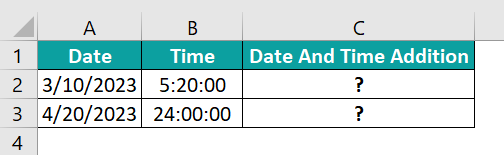And the requirement is to add the date and time value in each set and display the output in cells C2:C3. Then, the steps are as follows:

• Step 1: First, choose cell C2, enter the following formula, and press Enter.
=A2+B2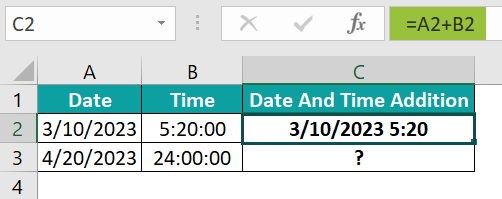• Step 2: Next, choose cell C3, enter the SUM(), and press Enter.
=SUM(A3+B3)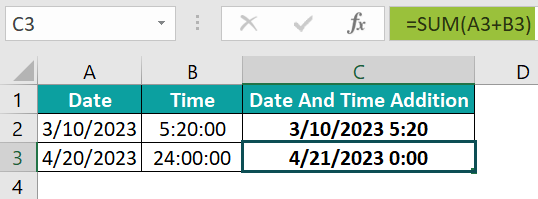In the first set, we need to add below 24 hours to the date. And hence, we see the specified date and time value as the final added value.

But we must add over 23 hours to the date value in the second set. And as 24 hours counts as a day, it increments the date by one. Also, the minutes and seconds in the time value are 00:00. Thus, adding the given date and time gives the output as 4/21/2023 0:00, indicating the 0:00 hour of the next day.

2. How do I add actual time in Excel?

We can add actual time in Excel by using the shortcut Ctrl + Shift + ; to enter the current time into a cell as the actual time. And then summing it with the given time value.

For example, the table below shows the first task time as 8:30.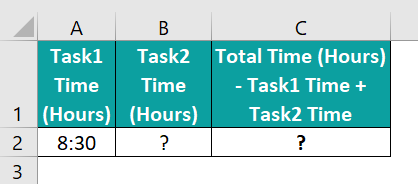Consider we finished the second task at the current time, which is the actual time. And we must find the total time taken to complete both tasks. Then, the steps are as follows:

• Step 1: First, select cell B2 and press the keys Ctrl + Shift + ; to enter the current or actual time in cell B2.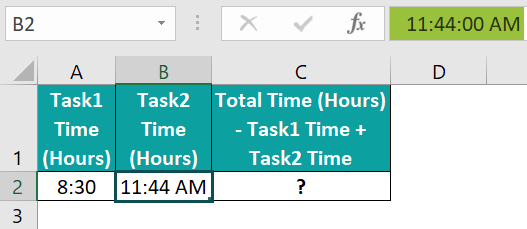• Step 2: Then, choose the target cell C2, enter the below formula to add the time values in cells A2 and B2, and press Enter.
=A2+B2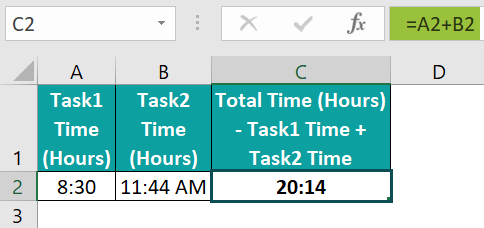3. Why can’t I add time in Excel?

We can’t add time in Excel, perhaps because of the following reasons:
The input time values are invalid.
The input time values are not in the required or correct time format.
The worksheet containing the input time values and where we are trying to add the time values is password-protected.